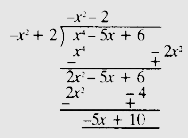# Divide the polynomial $p(x)$ by the polynomial $g(x)$ and find the quotient and remainder, in each of the following:$p(x) = x^4 - 5x + 6, g(x) = 2 -x^2$

Given:

$p(x) = x^4 - 5x + 6, g(x) = 2 -x^2$

To do:

We have to divide the polynomial $p(x)$ by the polynomial $g(x)$ and find the quotient and remainder.

Solution:

$p(x) = x^4 - 5x + 6$

$g(x) = 2 -x^2$Therefore, the quotient is $-x^2-2$ and the remainder is $-5x+10$.

Updated on: 10-Oct-2022

42 Views